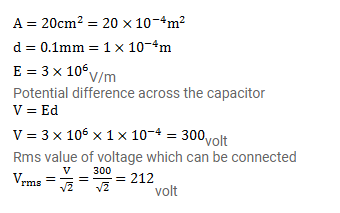# The dielectric strength of air is

Question:

The dielectric strength of air is $3.0 \times 10^{6} \mathrm{~V} / \mathrm{m}$. A parallel-plate air-capacitor has area $20 \mathrm{~cm}^{2}$ and plate separation $0.10 \mathrm{~mm}$. Find the maximum rms voltage of an AC source which can be safely connected to this capacitor.

Solution: